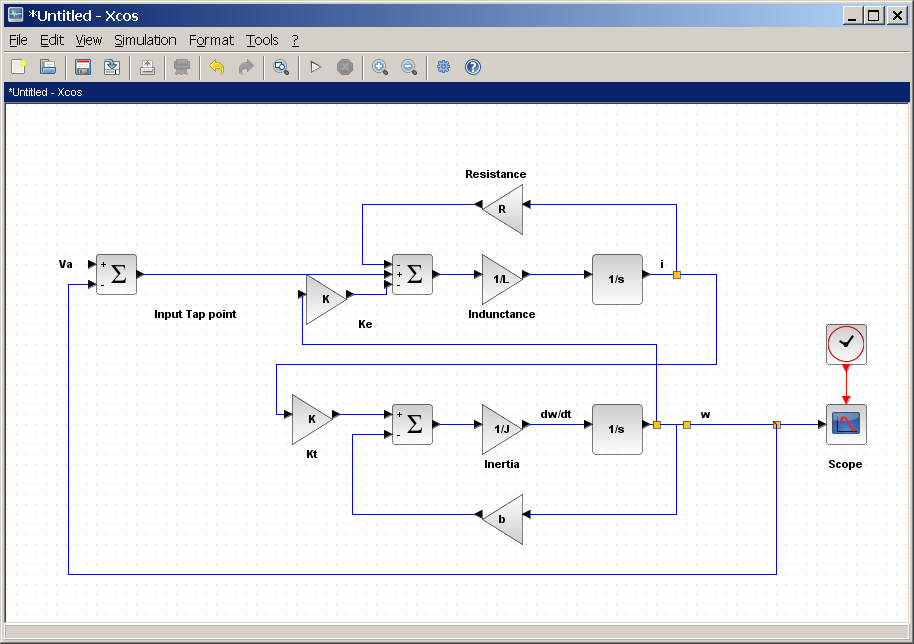# Equalis Data Acquisition and Control Systems in action

The Equalis Data Acquisition and Control Systems (DACS) module  allows a user to define a mathematical model of a typical control system in the form of a block diagram. From this model you can view critical charts and important characteristics of that system such as step response, Bode, Nyquist Nichols, Pole-Zero, and Singular values plots.In this example we use our Control and Power Systems module from DACS to analyze a linear model of a permanent magnet DC motor. This motor can be modeled as shown below.

Assuming a constant load, we can consider a DC motor as a SISO sysem, where the applied voltage Va is the input and the motor speed is the output. Using standard mathematical operations (such as summation, gain, integration), let us create a diagram of this SISO system.

The resulting diagram is shown below:The input Va can be connected to a constant block (this means a constant voltage).

The output w is the speed in rad/s.

Let us use Simulation>Set context to set the following values of constants which appear in the diagram:

J=3.2284E-6; b=3.5077E-6; K=0.0274; R=4; L=2.75E-6;

Now let us analyze the o